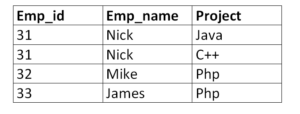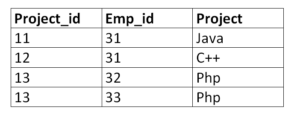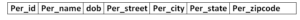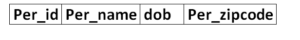# Database Normalization : A Basic IntroductionNormalization is a method of decomposing a set of relations (tables) that contain anomalies to produce smaller and well-structured relations with minimal redundancy. Basically, the rules of normalization are created to prevent data inconsistencies and update anomalies. The major objective of normalization is to reduce data redundancy, which means that the information or data should be stored only once. If the same data is stored several times, it may lead to a loss of data integrity, waste of server resources, and increases storage space requirements. The second main objective of normalization is to ensure data dependency, which means that the data should be stored logically.

General normalization objectives include:

• Minimize the need for restructuring a database when new data types are introduced.
• Make relations free from unwanted deletions, updates and insertions.

The rules of the normalization are divided into normal forms.

Normal Forms

When rules of functional dependencies are applied to relations, these relations are then transformed into a state that is known as Normal Form. Normalization checks that different kinds of inconsistencies are not introduced in the database. Different kinds of normal forms that are used in relational database management system (RDBMS) are:

1. First Normal form(1NF)
2. Second Normal form(2NF)
3. Third Normal form(3NF)
4. Boyce/Codd Normal form(BCNF)

First Normal form:

A relation is in the first normal form if the values that are in the domain of each attribute of relation are atomic. The first normal form forbids composite attributes, multi-valued attributes and any combinations. When it comes to an organized database, 1NF has two basic rules:

1. Remove identical columns from the same table.
2. Identify every row with a unique column or set of columns (primary key) and develop separate table for every group of related values.

Example:

Now, let’s take a table “employee” that is not in the first normal form in the following example.

employeeYou can see that the employee name “Nick” and project “PHP” are used twice in the table. This breaks the conditions of the first normal form. We divide this table into two distinct tables to convert it into first normal form.

New Employee TableProject TableNow both the employee table and the project table are in the first normal form.

Second Normal Form

The table is said to be in second normal form when it fulfills all the requirements of the first normal form and there should not be any partial dependencies of any column on the primary key. It means that each non-primary key attribute is completely dependent on the primary key of that relation. If any of the columns depend on only one part of a concatenated key, then the table does not fall into 2NF. Second normal form is an intermediate step to higher normal forms and it is based on complete functional dependency.

Example

Consider a table that is not in the second normal form.

ConsumerThe above table is in the first normal form but not in the second normal form. In this table, the concatenation of Con_id and Order_id is the primary key. This table is not in the first normal form, because partial dependencies are present on the primary key. Con_name is only dependent on Con_id and Order_name is only dependent on Order_id. There is no connection between Sale and Con_name. To convert this table into 2NF, you need to divide this table into three tables.

Consumer_detailSaleThe three tables above now fall into 2NF.

Third Normal Form

The table is said to be in the third normal form if it is in second normal form and :

1. Non–prime attributes should be mutually independent.
2. Non–prime attributes should be functionally dependent on primary key.

The relation in third normal form consists of a group of independent non-prime attributes and a primary key. In 3NF, it’s necessary to remove transitive functional dependencies from the table.

Example

Let’s take a table “person” for this example.

personIn the above table, Per_id is the primary key and Per_street, Per_city and Per_state depends on Per_zipcode. The dependencies between the Per_zipcode and other fields are known as transitive dependency. To convert the above table into 3NF, you need to place  Per_street, Per_city and Per_state into a new table with the Per_zipcode as the primary key.

Person_detailLearn about SQL Database from scratch by taking a course at Udemy.com

Boyce/Codd Normal form(BCNF):

Proposed by R.F. Boyce, the BCNF is marginally stronger than the third normal form (3NF) and is known as the Boyce/Codd Normal form. Basically, it is an extension of the 3NF and is therefore termed as the 3.5NF. Though the BCNF and 3NF are generally compliant, they can be non-compliant in rare cases when the tables contain candidate keys that overlap each other. However, the BCNF deals with anomalies in a better way that 3NF generally fails to handle.

We can say that a relation is in BCNF if the condition FD: XàY is true and the attributes X and Y holds in R. It can be written as:

1. X is the super key of R
2. X -> Y is trivial FD(Functional Dependency), where Y is a subset of X

The difference between 3NF and BCNF is that the primary key attribute is B while A is not the candidate key, the third normal form will allow it in a relation. However, in certain cases a functional dependency should be the candidate key and in such cases, the dependency belongs to the Boyce/Codd normal form (BCNF).

BCNF always acts differently from third normal form when multiple overlapping candidate keys are present. Unlike the above three normal forms, it is not always possible to achieve BCNF. There are some cases where you cannot convert the non-BCNF table into BCNF table. It is based on the concept of determinant, which is an attribute on which the other attributes are completely dependent.